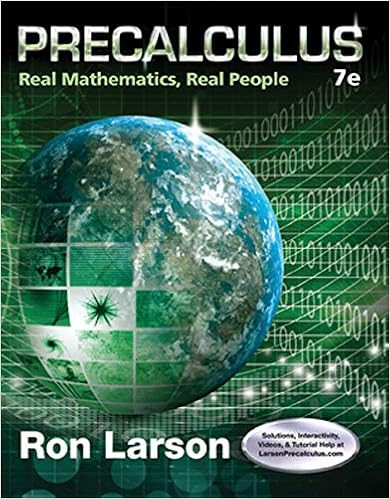# Real numbers and their properties 1 10 in general a

• Notes
• 84
• 100% (3) 3 out of 3 people found this document helpful

This preview shows page 10 - 12 out of 84 pages.

##### We have textbook solutions for you!
The document you are viewing contains questions related to this textbook.The document you are viewing contains questions related to this textbook.
Chapter 2 / Exercise 99
Precalculus: Real Mathematics, Real People
LarsonExpert Verified
Real Numbers and Their Properties 1-10 In general, a means “the opposite of a .” If a is positive, a is negative. If a is negative, a is positive. Opposites have the following property. Opposite of an Opposite For any real number a , ( a ) a . Absolute Value Remember that we have defined a to be the distance between 0 and a on the number line. Using opposites, we can give a symbolic definition of absolute value. a if a is positive or zero if a is negative a a E X A M P L E 7 Warm-Ups Fill in the blank. 1. The set of is {. . . , 3, 2, 1, 0, 1, 2, 3, . . .}. 2. The set of numbers is {1, 2, 3, . . .}. 3. Every number can be expressed as a ratio of integers. 4. and decimal numbers are rational numbers. 5. A decimal number that does not repeat and does not terminate is . 6. The rationals together with the irrationals form the set of numbers. 7. The ratio of the and diameter of any circle is . 8. The of a number is its distance form 0 on a number line. True or false? 9. The natural numbers and the counting numbers are the same. 10. Zero is a counting number. 11. Zero is an irrational number. 12. The opposite of negative 3 is positive 3. 13. The absolute value of 4 is 4. 14. The real number is in the interval (3, 4). 15. The interval (4, 9) contains 8. 16. The interval (2, 6) contains 6. 17. The interval [3, 5] contains 3. 18. The interval (9, ) contains 88 trillion. Using the symbolic definition of absolute value Evaluate. a) 8 b) 0 c) 8 Solution a) From the definition, a a if a is positive. Since 8 is positive, we replace a with 8 to get 8 8. b) From the definition, a a if a is zero. Replacing a with 0, we get 0 0. c) From the definition, a a if a is negative. Since 8 is negative, we replace a with 8 to get 8 ( 8) 8. Now do Exercises 55–60
##### We have textbook solutions for you!
The document you are viewing contains questions related to this textbook.The document you are viewing contains questions related to this textbook.
Chapter 2 / Exercise 99
Precalculus: Real Mathematics, Real People
LarsonExpert Verified
Exercises U Study Tips V Exercise sets are designed to increase gradually in difficulty. So start from the beginning and work lots of exercises. Find a group of students to work with outside of class. Explaining things to others improves your own understanding of the concepts. 1.1 U 3 V The Number Line Determine which number is the larger in each given pair of numbers. See Example 1. 1. 0, 6 2. 7, 4 3. 3, 6 4. 7, 10 5. 0, 6 6. 8, 0 7. 3, 2 8. 5, 8 9. 12, 15 10. 13, 7 11. 2.9, 2.1 12. 2.1, 2.9 List the numbers described and graph them on a number line. See Example 2. 13. The counting numbers smaller than 6 14. The natural numbers larger than 4 15. The whole numbers smaller than 5 16. The integers between 3 and 3 17. The whole numbers between 5 and 5 18. The integers smaller than 1 19. The counting numbers larger than 4 20. The natural numbers between 5 and 7 21. The integers larger than 1 2 22. The whole numbers smaller than 7 4 U 4 V The Real Numbers Determine whether each statement is true or false. Explain your answer. See Example 3. 23. Every integer is a rational number. 24. Every counting number is a whole number. 25. Zero is a counting number.
•••